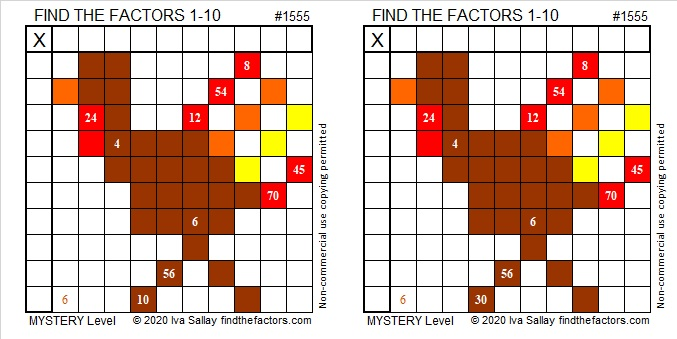# 1555 Two Turkeys Too Tough To Try?

### Today’s Puzzle:

Two turkeys too tough to try? That’s a six-word title made with alliteration and three homophones! It also describes the mystery-level turkey puzzles below. Those turkeys might look like identical twins at first glance, but if you look closely, you will see they are not quite the same.Here are some questions to help you find a logical way to start either puzzle: Which two clues MUST use the two 6’s as factors? Are there any other clues that are multiples of 6? If so, what factors would those clues use?

### Factors of 1555:

• 1555 is a composite number.
• Prime factorization: 1555 = 5 × 311.
• 1555 has no exponents greater than 1 in its prime factorization, so √1555 cannot be simplified.
• The exponents in the prime factorization are 1 and 1. Adding one to each exponent and multiplying we get (1 + 1)(1 + 1) = 2 × 2 = 4. Therefore 1555 has exactly 4 factors.
• The factors of 1555 are outlined with their factor pair partners in the graphic below.### Another Fact about the Number 1555:

1555 is the hypotenuse of a Pythagorean triple:
933-1244-1555, which is (3-4-5) times 311.